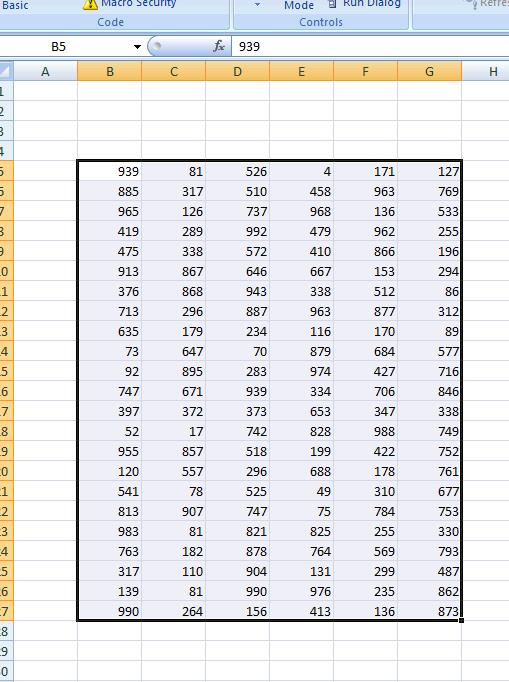# VBA – Find the Maximum Value For Each Column in a Range

## Find Max Value For Each Column in Range

The following function will return the Maximum Value in each Column in a Range:

``````Function Max_Each_Column(Data_Range As Range) As Variant
Dim TempArray() As Double, i As Long
If Data_Range Is Nothing Then Exit Function
With Data_Range
ReDim TempArray(1 To .Columns.Count)
For i = 1 To .Columns.Count
TempArray(i) = Application.Max(.Columns(i))
Next
End With
Max_Each_Column = TempArray
End Function``````

We can use a subroutine like the following to display the results:

``````Private Sub CommandButton1_Click()
Dim No_of_Cols As Integer
Dim i As Integer
No_of_Cols = Range("B5:G27").Columns.Count

For i = 1 To No_of_Cols
Next i
End Sub``````

So:Will return 990,907, 992, 976 ,988 and 873 for each of the above columns.
[SPECIAL THANKS TO MIKE RICKSON FOR RESOLVING THE FINERIES OF ARRAYS WITHIN UDF’S ]